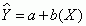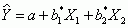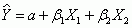# Chapter Main Points and Learning Objectives

Chapter main points

• A scatter diagram (also called scatterplot) is a quick visual method used to display relationships between two interval-ratio variables.
• Equations for all straight lines have the same general form:• The best-fitting regression line is that line where the residual sum of squares, or Σe2, is at a minimum. Such a line is called the least squares line, and the technique that produces this line is called the least squares method.
• The coefficient of determination (r2) and Pearson’s correlation coefficient (r) measure
• how well the regression model fits the data. Pearson’s r indicates the strength of the association between the two variables. The coefficient of determination is a PRE measure, identifying the reduction of error based on the regression model.
• The general form of the multiple regression equation involving two independent variables is. The multiple coefficient of determination (R2) measures the proportional reduction of error based on the multiple regression model.
• The standardized multiple regression equation is. The beta coefficients allow us to assess the relative strength of all the independent variables.

Learning objectives

1. Describe linear relationships and prediction rules for bivariate and multiple regression models
2. Construct and interpret straight-line graphs and best-fitting lines
3. Calculate and interpret a and b
4. Calculate and interpret the coefficient of determination (r2) and Pearson’s correlation coefficient (r)
5. Interpret SPSS multiple regression output
6. Test the significance of r2 and R2 using ANOVA